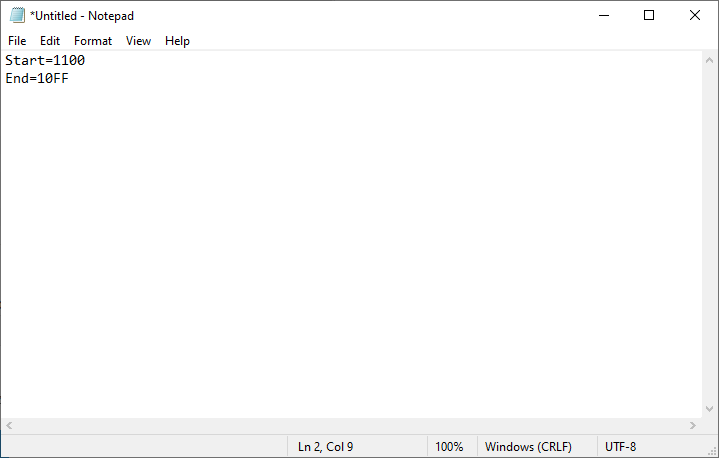# Check varbinary data

Nov 8 2020 8:13 PMI have to check the values of varbinary data in the text file. For example, in the text file, if the start range is 1100, while the end range is 10FF, it will continue to do the next process, else it will prompt the range is not meet the end range yet.

This is my current code:
1. var data = File
3.  .Select(x => x.Split('='))
4. .Where(x => x.Length > 1)
5.  .ToDictionary(x => x.Trim(), x => x);
6.
7. Console.WriteLine("Start: {0}", data["Start"]);
8. Console.WriteLine("End: {0}", data["End"]);
9.
10. if (data["Start"] == data["End"]+1)
11. {
12.       //it will proceed to next process
13. }
14. else
15. {
16.     //prompt not meet end range
17. }
This is the text fileThe problem is, the if (data["Start"] == data["End"]+1) does not functioning. any other method that I can do?